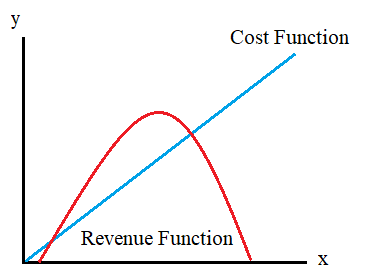# Cost Revenue and Profit Function Examples

Cost, revenue and profit functions are three very useful functions that can help you evaluate a businesses or organization’s success (or failure). Cost revenue and profit function examples typically describe the relationship between:

• Quantity (or volume) produced (or sold),
• The costs a company incurs,
• Generated revenue,
• Overall profits.Each function has its own particular characteristics.

## Revenue Function Cost Function ProfitFunction 1. Calculating the Revenue Function

If one type of product is being sold at one price, the revenue function is simply:

R = p x

Where:

• R = revenue,
• p = price per unit,
• x = number of units sold.

For example, if a lemonade stand sold x glasses of lemonade at 50 cents each, the revenue function would be

R = \$0.50 x.

When more than one item is sold, or different prices are used, new terms must be added to the revenue function. The function always keeps the form

R = p1x1 + p2x2 + … +pnxn
Where:

• pi is the price for the item,
• xi is the number of items sold.

If the lemonade stand also sold cookies for \$1 apiece, the revenue function would be

Where:

## 2. Calculating the Cost Function

Even in a simple situation where only one item is sold, a cost function typically has two components:

1. Base cost: the amount of overhead that doesn’t depend on how much of an item is manufactured/sold. This would include the cost of the stand, the juicer, and the jugs and cups which are a one-time buy.
2. Unit cost: The number of items manufactured or sold times what it cost to make each item.

So we have:

C = base_cost + unit_cost · x.

If it costs \$50 to buy what we need to start a lemonade stand, and 10 cents of materials for each cup, the cost function for that situation is

C = \$50.0 + \$0.10 x

Just as we did for the revenue function, we will need to add terms for additional items that are being manufactured. If every cookie cost 50 cents to make, our revenue function becomes

Fun fact: Until recently, econometric cost functions were considered too technical for courts to understand. This changed when a court case in Missouri featured cost-function estimates .

## Calculating the Profit Function

The profit function is just the revenue function minus the cost function. We can write

Profit = R – C

For our simple lemonade stand, the profit function would be

Profit = (\$0.50 x)-(\$50.00 + \$0.10 x)

= \$0.40 x – \$50.00

This function is extremely useful, it can tell us, for example, how many glasses of lemonade we would need to sell to break even. In this case, that would be 50/0.40, or 125.

## Cost Revenue and Profit Function Examples References

 Costrell, H. & Loeb, S. (2008). What Do Cost Functions Tell Us About the Cost of an Adequate Education? Peabody Journal of Education, 83: 198-223.

CITE THIS AS:
Stephanie Glen. "Cost Revenue and Profit Function Examples" From StatisticsHowTo.com: Elementary Statistics for the rest of us! https://www.statisticshowto.com/cost-revenue-and-profit-function-examples/

### 4 thoughts on “Cost Revenue and Profit Function Examples”

1. this is good

2. Can you help me with these??
You are an aspiring entrepreneur who wants to put up a T-shirt business. You are tasked to make a proposal that contains the following, among others:

1. a formula that will represent your profit as a function of cost.
Determine the number of T-shirts where you will have a break even, a loss, and a profit .
2. A table of values and a graph
3. The initial capital you will be needing to start off the business.

I can’t really understand these kind of things, can you help me?

3. Where do you get stuck?

4. 1. A company produces x items at a fixed cost of shs 24 and variable cost of shs 4 per unit. If the items are sold at shs 8 per unit, find the
(i) revenue function
(ii) cost function
(iii) break even quantity